# Samacheer Kalvi Books: Tamilnadu State Board Text Books Solutions

## Samacheer Kalvi 10th Science Chapter 9 Solutions Notes PDF Download: Tamil Nadu STD 10th Science Chapter 9 Solutions NotesSamacheer Kalvi 10th Science Chapter 9 Solutions Notes PDF Download: Tamil Nadu STD 10th Science Chapter 9 Solutions Notes

We bring to you specially curated Samacheer Kalvi 10th Science Chapter 9 Solutions Notes PDF which have been prepared by our subject experts after carefully following the trend of the exam in the last few years. The notes will not only serve for revision purposes, but also will have several cuts and easy methods to go about a difficult problem.

 Board Tamilnadu Board Study Material Notes Class Samacheer Kalvi 10th Science Subject 10th Science Chapter Chapter 9 Solutions Format PDF Provider Samacheer Kalvi Books

## How to Download Samacheer Kalvi 10th Science Chapter 9 Solutions Notes PDFs?

2. Click on the Samacheer Kalvi 10th Science Notes PDF.
3. Look for your preferred subject.
4. Now download the Samacheer Kalvi 10th Science Chapter 9 Solutions notes PDF.

## Download Samacheer Kalvi 10th Science Chapter 9 Solutions Chapterwise Notes PDF

### Samacheer Kalvi 10th Science Solutions Textual Problems Solved

I. Problems based on Solubility.

10th Science Samacheer Kalvi Question 1.
1.5 g of solute is dissolved in 15 g of water to form a saturated solution at 298K. Find out the solubility of the solute at the temperature.
Solution:
Mass of the solute = 1.5 g
Mass of the solvent = 15 g
Solubility of the solute =  Mass of the solute  Mass of the solvent ×100
= 1.515×100=10g.

Samacheer Kalvi 10th Science Question 2.
Find the mass of potassium chloride would be needed to form a saturated solution in 60 g of water at 303 K? Given that solubility of the KCl is 37 / 100 g at this temperature.
Solution:
Mass of potassium chloride in 100 g of water in saturated solution = 37 g
Mass of potassium chloride in 60 g of water in saturated solution = 37100×60 = 22.2 g.

Samacheer Kalvi Class 10 Science Solutions Question 3.
What is the mass of sodium chloride that would be needed to form a saturated solution in 50 g of water at 30°C. Solubility of sodium chloride is 36 g at 30°C?
Solution:
At 30°C, 36 g of sodium chloride is dissolved in 100 g of water.
Mass of sodium chloride that would be need for 100 g of water = 36 g
Mass of sodium chloride dissolved in 50 g of water = 36×50100 = 18 g.

10th Samacheer Kalvi Science Question 4.
The Solubility of sodium nitrate at 50°C and 30°C is 114 g and 96 g respectively. Find the amount of salt that will be thrown out when a saturated solution of sodium nitrate-containing 50 g of water is cooled from 50°C to 30°C?
Amount of sodium nitrate dissolved in 100 g of water at 50°C is 114 g
Amount of sodium nitrate dissolving in 50 g of water at 50°C is = 114×50100 = 57 g
Similarly amount of sodium nitrate dissolving in 50 g of water at 30°C is = 96×50100 = 48 g
Amount of sodium nitrate thrown when 50 g of water is cooled from 50°C to 30°C is 57 – 48 = 9 g.

II. Problems based on Mass percentage.

Samacheer Kalvi 10th Science Solutions Question 1.
A solution was prepared by dissolving 25 g of sugar in 100 g of water. Calculate the mass percentage of solute.
Solution:
Mass of the solute = 25 g
Mass of the solvent = 100 g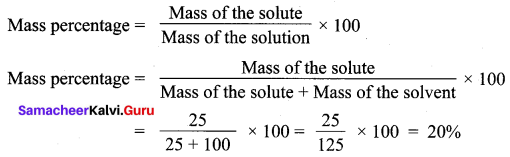Class 10 Science Samacheer Kalvi Question 2.
16 grams of NaOH is dissolved in 100 grams of water at 25°C to form a saturated solution. Find the mass percentage of solute and solvent.
Solution:
Mass of the solute (NaOH) = 16 g
Mass of the solvent H2O = 100 g
(i) Mass percentage of the solute
=  Mass of the solute  Mass of the solute + Mass of the solvent ×100
= 16×10016+100=1600116
Mass percentage of the solute = 13.79 %.

(ii) Mass percentage of solvent = 100 – (Mass percentage of the solute) = 100 – 13.79 = 86.21 %.

Science Solution Class 10 Samacheer Kalvi Question 3.
Find the amount of urea which is to be dissolved in water to get 500 g of 10 % w/w aqueous solution?
Solution:
Mass percentage =  Mass of the solute  Mass of the solution ×100
10= Mass of the urea 500×100
Mass of urea = 50 g.

III. Problem based on Volume – Volume percentage.

Samacheer Kalvi 10th Science Book Solutions Question 1.
The solution is made from 35 ml of methanol and 65 ml of water. Calculate the volume percentage.
Solution:
The volume of the ethanol = 35 ml
The volume of the water = 65 ml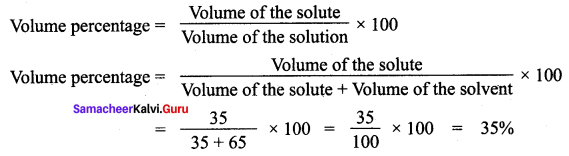10th Science Solution Samacheer Kalvi Question 2.
Calculate the volume of ethanol in 200 ml solution of 20 % v/v aqueous solution of ethanol.
Solution:
Volume of aqueous solution = 200 ml
Volume percentage = 20 %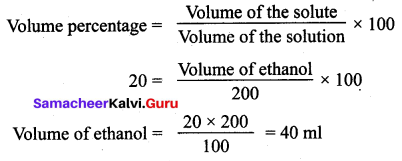Activity

10th Std Science Solutions Samacheer Kalvi Activity 1.
Look at the following pictures. Label them as a dilute and concentrated solution and justify your answer.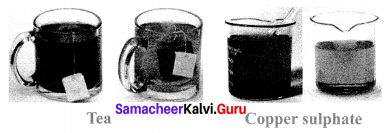Solution:
In the above picture, the first teacup is more concentrated and the first beaker is more concentrated CuSO4 solution. The reason is the colour intensity is more in a concentrated solution.

### Samacheer Kalvi 10th Science Solutions Textual Evaluation Solved

Class 10 Science Solutions Samacheer Kalvi Question 1.
A solution is a ____ mixture.
(a) homogeneous
(b) heterogeneous
(c) homogeneous and heterogeneous
(d) non – homogeneous.
(a) homogeneous

Science 10th Samacheer Kalvi Question 2.
The number of components in a binary solution is:
(a) 2
(b) 3
(c) 4
(d) 5
(a) 2

Samacheer Kalvi 10th Science Answers Question 3.
Which of the following is the universal solvent?
(a) Acetone
(b) Benzene
(c) Water
(d) Alcohol.
(c) Water

Science Class 10 Samacheer Kalvi Question 4.
A solution in which no more solute can be dissolved in a definite amount of solvent at a given temperature is called:
(a) Saturated solution
(b) Unsaturated solution
(c) Supersaturated solution
(d) Dilute solution
(a) Saturated solution

Samacheer Class 10 Science Solutions Question 5.
Identify the non – aqueous solution ______.
(a) sodium chloride in water
(b) glucose in water
(c) copper sulphate in water
(d) sulphur in carbon-di-sulphide.
(d) sulphur in carbon-di-sulphide.

Samacheer Kalvi Guru 10th Science Question 6.
When pressure is increased at a constant temperature the solubility of gases in liquid:
(a) No change
(b) increases
(c) decreases
(d) no reaction
(b) increases

Science Samacheer Kalvi Question 7.
The solubility of NaCl in 100 ml water is 36 g. If 25 g of salt is dissolved in 100 ml of water how much more salt is required for saturation ______.
(a) 12 g
(b) 11 g
(c) 16 g
(d) 20 g.
(b) 11 g

Samacheer Kalvi 10 Science Question 8.
A 25% alcohol solution means:
(a) 25 ml alcohol in 100 ml of water
(b) 25 ml alcohol in 25 ml of water
(c) 25 ml alcohol in 75 ml of water
(d) 75 ml alcohol in 25 ml of water
(c) 25 ml alcohol in 75 ml of water

Deliquescence is due to ______.
(a) Strong affinity to water
(b) Less affinity to water
(c) Strong hatred of water
(d) Inertness to water.
(a) Strong affinity to water

Samacheer Kalvi.Guru 10th Science Question 10.
Which of the following is hygroscopic in nature?
(a) ferric chloride
(b) copper sulphate penta hydrate
(c) silica gel
(d) none of the above
(c) silica gel

II. Fill in the blanks.

10th Standard Science Samacheer Kalvi Question 1.
The component present in a lesser amount, in a solution, is called ______.
Solute.

Samacheer Kalvi 10th Standard Science Question 2.
Example for liquid in solid type solution is ______.
Sodium chloride dissolved in water.

Question 3.
Solubility is the amount of solute dissolved in _______ g of solvent.
100.

Question 4.
Polar compounds are soluble in ______ solvents.
Polar.

Question 5.
Volume percentage decreases with increases in temperature because of ______.
Expansion of liquid.

III. Match the following.

Question 1.

 Blue vitriol (a) CaSO4.2H2O Gypsum (b) CaO Deliquescence (c) CuSO4.5H2O Hygroscopic (d) NaOH

1 – (c), 2 – (a), 3 – (d), 4 – (b).

IV. True or False: (If false give the correct statement)

Question 1.
Solutions which contain three components are called binary solution.
False.
Correct Statement: Solutions which contain two components are called binary solution.

Question 2.
In a solution, the component which is present in a lesser amount is called solvent.
False.
Correct Statement:

• In a solution, the component which is present in a larger amount is called a solvent.
• In a solution, the component which is present in a lesser amount is called solute.

Question 3.
Sodium chloride dissolved in water forms a non-aqueous solution.
False.
Correct Statement: Sodium chloride dissolved in water forms an aqueous solution.

Question 4.
The molecular formula of green vitriol is MgSO4.7H2O
False.
Correct Statement: The molecular formula of green vitriol is FeSO4 .7H2O
Question 5.
When Silica gel is kept open, it absorbs moisture from the air, because it is hygroscopic in nature.
True.

Question 1.
Define the term Solution.
A solution is a homogeneous mixture of two or more substances.

Question 2.
What is mean by binary solution?
Solutions which are made of one solute and one solvent, then it is called binary solution.

Question 3.
Give an example each

1. gas in liquid
2. solid in liquid
3. solid in solid
4. gas in gas.

1. Carbon – di – oxide dissolved in water (Soda water).
2. Sodium chloride dissolved in water.
3. Copper dissolved in gold (Alloy).
4. A mixture of Helium – Oxygen gases.

Question 4.
What is aqueous and non-aqueous solution? Give an example.
(i) Aqueous solution : The solution in which water acts as a solvent.
(ii) Non-aqueous solution : The solution in which any liquid other than water acts as a solvent.
Eg: Alcohol, benzene, CS2 acetone.

Question 5.
Define Volume percentage.
Volume percentage is defined as the percentage by volume of solute (in ml) present in the given volume of the solution.
Volume percentage =  Volume of the solute  Volume of the solution ×100.

Question 6.
The aquatic animals live more in cold region Why?
Aquatic animals live more in cold regions because the solubility of O2 in water is more at low temperature and therefore the amount of dissolved O2 is more in the water of cold regions.

Question 7.
Define Hydrated salt.
The number of water molecules found in the crystalline substance or salts is called water of crystallization. Such salts are called hydrated salts.

Question 8.
A hot saturated solution of copper sulphate forms crystals as it cools. Why?
A hot saturated solution of CuSO4 forms crystal as it cools. Because on cooling the water molecules move closer together and there is less space for the solution to hold on to as much of the dissolved solid and so it forms crystals.

Question 9.
Classify the following substances into deliquescent, hygroscopic. Conc. Sulphuric acid, Copper sulphate penta hydrate, Silica gel, Calcium chloride, and Gypsum salt.

1. Deliquescent substances: Calcium chloride
2. Hygroscopic substances: Conc Sulphuric acid, Copper sulphate penta hydrate, Silica gel and Gypsum salt.

Question 1.
Write notes on
(i) saturated solution
(ii) unsaturated solution
(i) Saturated solution : A solution in which no more solute can be dissolved in a definite amount of the solvent at a given temperature is called saturated solution.
Eg: 36 g of sodium chloride in 100 g of water at 25°C forms saturated solution.

(ii) Unsaturated solution : Unsaturated solution is one that contains less solute than that of the saturated solution at a given temperature.
Eg: 10 g or 20 g or 30 g of Sodium chloride in 100 g of water at 25°C forms an unsaturated solution.

Question 2.
Write notes on various factors affecting solubility.
Factors affecting solubility: There are three main factors which govern the solubility of the solute. They are
(i) Nature of the solute and solvent
(ii) Temperature
(iii) Pressure

(i) Nature of the solute and solvent: The nature of the solute and solvent plays an important role insolubility. Although water dissolves an enormous variety of substances, both ionic and covalent, it does not dissolve everything. The phrase that scientists often use when predicting solubility is “like dissolves like.” This expression means that dissolving occurs when similarities exist between the solvent and the solute. For example, Common salt is a polar compound and dissolves readily in polar solvent like water.

Non – polar compounds are soluble in non-polar solvents. For example, Fat dissolved in ether. But non-polar compounds, do not dissolve in polar solvents; polar compounds do not dissolve in non-polar solvents.

(ii) Effect of Temperature
The solubility of Solids in Liquid: Generally, the solubility of a solid solute in a liquid solvent increases with increase in temperature. For example, a greater amount of sugar will dissolve in warm water than in cold water.
In the endothermic process, solubility increases with increase in temperature. In the exothermic process, solubility decreases with increase in temperature.

The solubility of Gases in liquid: Solubility of gases in liquids decreases with increase in temperature. Generally, water contains dissolved oxygen. When water is boiled, the solubility of oxygen in water decreases, so oxygen escapes in the form of bubbles. Aquatic animals live more in cold regions because more amount of dissolved oxygen is present in the water of cold regions. This shows that the solubility of oxygen in water is more at low temperatures.

(iii) Effect of Pressure: Effect of pressure is observed only in the case of solubility of a gas in a liquid. When the pressure is increased, the solubility of a gas in liquid increases.
The common examples for solubility of gases in liquids are carbonated beverages, i.e. soft drinks, household cleaners containing an aqueous solution of ammonia, formalin aqueous solution of formaldehyde, etc.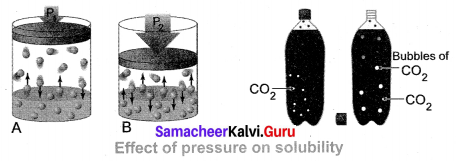Question 3.
(a) What happens when MgSO4.7H2O is heated? Write the appropriate equation.
(b) Define solubility.
(a) MgSO4.7H2O has a water of crystallization is 7. When magnesium sulphate heptahydrate crystals are gently heated, it loses seven water molecules and becomes anhydrous magnesium sulphate.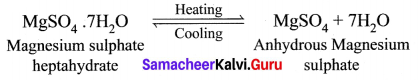(b) Solubility: It is defined as the number of grams of a solute that can be dissolved in 100 g of a solvent to form its saturated solution at a given temperature and pressure.

Question 4.
In what way, hygroscopic substances differ from deliquescent substances.
Difference between hygroscopic and deliquescent substances is in the extent to which each material can absorb moisture. This is because both of these terms are very much related to each other and they refer to the property of observing and the retention of moisture from the air. However, they differ in the extent of absorption of moisture where hygroscopic materials absorb moisture but not to the extent the original substance dissolves in it, which is the case with deliquescence. Therefore deliquescence can be regarded as an extreme condition of hygroscopic activity.

Difference between hygroscopic substances and deliquescence

 Hygroscopic Substances Deliquescence Substances When exposed to the atmosphere at ordinary temperature, they absorb moisture and do not dissolve. When exposed to the atmospheric air at ordinary temperature, they absorb moisture and dissolve. Hygroscopic substances do not change their physical state on exposure to air. Deliquescent substances change its physical state on exposure to air. Hygroscopic substances may be amorphous solids or liquids. Deliquescent substances are crystalline solids.

Question 5.
A solution is prepared by dissolving 45 g of sugar in 180 g of water. Calculate the mass percentage of solute.
Mass of sugar (solute) = 45 g
Mass of water (solvent) = 180 g.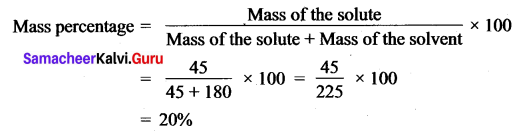Question 6.
3.5 litres of ethanol is present in 15 litres of an aqueous solution of ethanol. Calculate volume per cent of the ethanol solution.
Volume of ethanol (solute) = 3.5 litre
Volume of aqueous solution = 15 litre
Volume of the solution =  Volume of the solute  Volume of the solution ×100
= 3.515×100
Volume percentage = 23.33 %.

VII. HOT Questions.

Question 1.
Vinu dissolves 50 g of sugar in 250 ml of hot water, Sarath dissolves 50 g of same sugar in 250 ml of cold water. Who will get faster dissolution of sugar? and Why?
Vinu will get faster dissolution of sugar. Because Vinu dissolves 50 g of sugar in 250 ml of hot water, whereas Sarath dissolves 50 g of sugar in 250 ml of cold water. Solubility of a solid in liquid increases with increase in temperature.

Question 2.
‘A’ is a blue coloured crystalline salt. On heating, it loses a blue colour and to give ‘B’. When water is added, ‘B’ gives back to ‘A’. Identify A and B, write the equation.

• Blue coloured crystalline salt is copper sulphate pentahydrate (A)
• On heating Copper sulphate pentahydrate it loses blue colour and to give anhydrous copper sulphate (B).
• When water is added to the anhydrous copper sulphate (B) gives back to copper sulphate pentahydrate (A).
•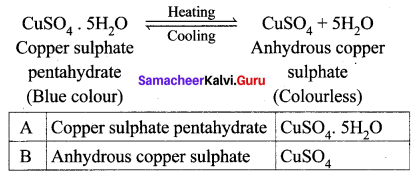Question 3.
Will the cool drinks give more fizz at top of the hills or at the foot? Explain.
Carbonated cool drinks give more fizz at the foot hill because solubility of gases in liquid decrease with increase in temperature. At higher altitudes, the temperature is low and the dissolved CO2 will not escape as fizz, whereas at the foot hill it does.

### Samacheer Kalvi 10th Science Solutions Additional Questions Solved

Question 1.
Find out the homogeneous mixture.
(a) Salt + Water
(b) Sand + Water
(c) Clay + Water
(d) Gold + Water.
(a) Salt + Water

Question 2.
The aqueous solution is:
(a) S in CS2
(b) I2 in CCl4
(c) Salt in H2O
(d) None of the above
(c) Salt in H2O

Question 3.
In a solution, the component which is present in a larger amount is called ______.
(a) solvent
(b) dissolution
(c) solute
(d) mole.
(а) solvent

Question 4.
The solubility of ammonia gas at 25°C is:
(a) 36 g
(b) 48 g
(c) 80 g
(d) 184 g
(b) 48 g

Question 5.
Example for liquid in liquid binary solution is ______.
(a) Copper dissolved in gold
(b) Water vapour in the air
(c) Ethyl alcohol dissolved in water
(d) NaCl dissolved in water.
(c) Ethyl alcohol dissolved in water

Question 6.
Which one of the following is an aqueous solvent?
(a) Benzene
(b) Acetone
(c) Alcohol
(d) Water.
(d) Water.

Question 7.
Mass percentage of a solution is independent of:
(a) temperature
(b) amount of solute
(c) amount of solvent
(d) chemical nature of the solute
(a) temperature

Question 8.
Solubility is equal to ______.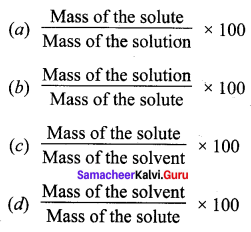(c)  Mass of the solute  Mass of the solvent ×100

Question 9.
In which case solubility increases with increase in temperature?
(a) Endothermic process
(b) Exothermic process
(c) Both (a) and (b)
(b) None of these.
(a) Endothermic process

Question 10.
The factor/factors which affect the solubility of a solute:
(a) Nature of the solute and solvent
(b) Temperature
(c) Pressure
(d) All of these
(d) All of these

Question 11.
The effect of pressure on the solubility of a gas in a liquid is given by ______.
(a) Hess’s law
(b) Ohm’s law
(c) Henry’s law
(d) Gases law.
(c) Henry’s law

Question 12.
5% sugar solution means ______.
(a) 5 g of sugar in 95 g of water
(b) 50 g of sugar in 50 g of water
(c) 20 g of sugar in 80 g of water
(d) 95 g of sugar in 5 g of water.
(a) 5 g of sugar in 95 g of water

Question 13.
The water of crystallisation present in white vitriol is:
(a) 2
(b) 7
(c) 5
(d) 24
(b) 7

Question 14.
Mass percentage is independent of ______.
(a) density
(b) volume
(c) pressure
(d) temperature.
(d) temperature.

Question 15.
Volume percentage is expressed as ______.
(a) v/v
(b) w/w
(c) v/w
(d) w/v.
(a) v/v

Question 16.
When the temperature increases, volume percentage ______.
(a) Increases
(b) Decreases
(c) no change
(d) increases then decrease.
(b) Decreases

Question 17.
The mass of NaCl needed to form saturated solution in 50 g of water at 30°C. When the solubility of NaCl is 36 g at 30°C is:
(a) 9 g
(b) 18 g
(c) 57 g
(d) 19 g
(b) 18 g

Question 18.
Zinc sulphate heptahydrate is also called as ______.
(a) Blue Vitriol
(b) Epsom salt
(c) Green Vitriol
(d) White Vitriol.
(d) white Vitriol.
Question 19.
Water of crystallization of blue vitriol is ______.
(a) 1
(b) 5
(c) 4
(d) 2.
(b) 5

Question 20.
On heating copper sulphate pentahydrate crystals ______.
(a) blue colour changes into green
(b) green colour changes into blue
(c) blue colour changes into colourless
(d) no colour changes.
(c) blue colour changes into colourless

Question 21.
Water of crystallization of Epsom salt is ______.
(a) 1
(b) 5
(c) 4
(d) 1.
(d) 7.

Question 22.
Hygroscopic substances are used as ______.
(a) drying agents
(b) hydrating agents
(c) freezing agents
(d) reducing agents.
(a) drying agents

Question 23.
If two liquids are mutually soluble, they are called _____ liquids.
(a) miscible
(b) immiscible
(c) insoluble
(d) viscous.
(a) miscible

Question 24.
Aquatic species are more comfortable in cold water because of ______.
(a) as the temperature decreases, the solubility of dissolved oxygen increases
(b) as the temperature increases, the solubility of dissolved oxygen increases
(c) as the temperature increases, the solubility of dissolved oxygen decreases
(d) None of these.
(a) as the temperature decreases, the solubility of dissolved oxygen increases

Question 25.
The process of food assimilation by man is in the form of ______.
(a) solution
(b) solid
(c) solute
(d) solvent.
(a) solution

Question 26.
Common salt in water is an example of ______.
(a) Colloidal solution
(b) Binary solution
(c) Suspension
(d) all the above.
(b) Binary solution

Question 27.
36 g of NaCl in 100 g of water is a ____ solution.
(a) Non – aqueous
(b) Unsaturated
(c) Supersaturated
(d) Saturated.
(d) Saturated.
Question 28.
Nitrogen in soil is an example of _____ solution in nature.
(a) saturated
(b) unsaturated
(c) supersaturated
(d) aqueous.
(a) saturated

Question 29.
The solubility of CuSO4 in H2O is ______ at 20°C.
(a) 36 g
(b) 20.7 g
(c) 10 g
(d) 95 g.
(b) 20.7 g

Question 30.
The solubility of NaNO3 in 100 g water at 25°C is ______.
(a) 36 g
(b) 95 g
(c) 184 g
(d) 92 g.
(d) 92 g.

Question 31.
Which of the following affect solubility?
(a) Temperature
(b) Nature of solute and solvent
(c) Pressure
(d) all the above.
(d) all the above.

Question 32.
In _____ process, solubility increases with increase in temperature.
(a) exothermic
(b) endothermic
(c) isothermic
(b) endothermic

Question 33.
In ______ process, solubility decreases with increase in temperature.
(a) exothermic
(c) endothermic
(d) isothermic.
(a) exothermic

Question 34.
Increase in ______ increases the solubility of gases.
(a) temperature
(b) pressure
(c) no. of moles
(d) concentration.
(b) pressure

Question 35.
The solubility of NaBr in H2O is ______.
(a) 36 g
(b) 95 g
(c) 184 g
(d) 92 g.
(b) 95 g

Question 36.
The gas – filled in soft drinks in ______.
(a) O2
(b) N2
(c) CO2
(d) H2
(c) CO2

Question 37.
The solubility of KNO3 in water is ______ process.
(a) Exothermic
(b) endothermic
(c) Isothermic
(b) endothermic

Question 38.
The solubility of CaO in water is ______ process.
(a) Exothermic
(b) endothermic
(d) Isothermic.
(a) Exothermic

Question 39.
The solubility of NaI in water is ______.
(a) 184 g
(b) 92 g
(c) 95 g
(d) 36 g.
(a) 184 g

II. Fill in the blanks.

Question 1.
A solution is a _____ mixture of two or more substances.
Homogeneous.

Question 2.
Common salt dissolved in water is an example for _____ solution.
Binary.

Question 3.
In a solution, the component present in a lesser amount by weight is called ____ and in a large amount by weight is called ______.
Solute, solvent.

Question 4.
The solution in which water acts as a solvent is called ______ and the solution in which Benzene acts as a solvent is called ______.

1. The aqueous solution,
2. Non – aqueous solution.

Question 5.
20 g of NaCl in 100 g of water is _____ solution.
Unsaturated.

Question 6.
36 g of NaCl in 100 g of water at room temperature forms a ____ solution.
Saturated.

Question 7.
Nitrogen in soil is an example for _____ in nature.
Saturated solution.

Question 8.
A solution which has more of solute than the saturated solution at a given temperature is called ______ solution.
Supersaturated.

Question 9.
In _____ process, solubility increases with ______ in temperature.
Endothermic, increase.

Question 10.
In an ______ process, solubility decreases with ______ in temperature.
Exothermic, increase.

Question 11.
The solubility of KNO3 increases with the _____ in temperature.
Increase.

Question 12.
The solubility of CaO decreases with the ______ in temperature.
Increase.

Question 13.
The solubility of oxygen is _____ in cold water.
More.

Question 14.
A polar compound is insoluble in _____ solvent.
Non – polar.

Question 15.
An increase in ______ increases the solubility of a gas in a liquid.
Pressure.

Question 16.
______ gas is filled in soft drinks using the effect of pressure.
CO2

Question 17.
Hygroscopic substances are used as ______.
Drying agents.

Question 18.
Anhydrous calcium chloride is an example of ______.
hygroscopic substance.

Question 19.
Hygroscopic substances absorb moisture without changing their ______.
Physical state.

Question 20.
Deliquescent substances lose their ______.
Crystalline shape.

Question 21.
Ferric chloride is an example of ______.
Deliquescent substances.

Question 22.
On heating, hydrated crystalline salts lose their ______.
Water of crystallization.

Question 23.
The number of water molecules in blue vitriol is ______.
Five.

Question 24.
The number of water molecules in magnesium sulphate heptahydrate is ______.
Seven.

Question 25.
Mass percentage is _______ to temperature.
Independent.

Question 26.
To qualify the solute in a solution, we can use the term ______.
Concentration.

Question 27.
Based on the amount of solute, the solution is classified into ________ types.
Three.

Question 28.
Zinc dissolved in copper is an example of ______ solution.
Solid in solid.

Question 29.
______ is an example of gas in the liquid.
Carbon dioxide dissolved in water.

Question 30.
______ and _______ are in the form of solution to decide the physiological activity of human beings.
Blood, Lymph.

III. Match the following.

Question 1.

 i. Sugar in water (a) Exothermic process ii. Sulphur in carbon sulphide (b) Aqueous solution iii. The solubility of CaO (c) Endothermic process iv. Solubility of KNO3 (d) Non-aqueous solution

i – b, ii – d, iii – a, iv – c.

Question 2.

 i. Saturated solution (a) Sulphur in acetone ii. Unsaturated solution (b) 56 g NaCl in water iii. Supersaturated solution (c) 10 g of NaCl in water iv. Non-aqueous solution (d) 36 g of NaCl in water

i – d, ii – c, iii – b, iv – a.

Question 3.

 i. Solubility of KNO3 (a) decreases with increase in temperature ii. The solubility of CaO (b) increases with increase in pressure iii. The solubility of oxygen (c) increases with increase in temperature iv. Solubility of CO2 (d) more in cold water

i – c, ii – a, iii – d, iv – b.

Question 4.

 i. Mercury with sodium (a) Liquid in gas ii. NaCl in water (b) Liquid in solid iii. CO2 in water (c) Solid in liquid iv. Water vapour in air (d) Gas in liquid

i – b, ii – c, iii – d, iv – a.

Question 5.

 Solute Solubility i. CaCO3 (a) 80 ii. NH3 (b) 91 iii. NaOH (c) 0.0013 iv. C6H12O6 (d) 48

i – c, ii – d, iii – a, iv – b.

Question 6.

 i. Epsom salt (a) ZnSO4.7H2O ii. Green Vitriol (b) MgSO4.7H2O iii. White Vitriol (c) SiO2 iv. Silica gel (d) FeSO4.7H2O

i – b, ii – d, iii – a, iv – c.

IV. State whether true or false. If false, give the correct statement.

Question 1.
A solution is a heterogeneous mixture of two or more substances.
False.
Correct statement: A solution is a homogeneous mixture of two or more substances.

Question 2.
A solution contains four components, it is called as a binary solution.
False.
Correct statement: A solution contains two components, it is called as a binary solution.

Question 3.
The solution in which water acts as a solvent, it is called a non-aqueous solution.
False.
Correct statement: The solution in which water acts as a solvent, it is called an aqueous solution.

Question 4.
The solution of sulphur in CS2 is a suitable example of a non-aqueous solution.
True.

Question 5.
Nitrogen in soil is an example of a supersaturated solution.
False.
Correct statement: Nitrogen in the soil is an example of a saturated solution.

Question 6.
The solubility of CuSO4 in H2O is 36 g at 25°C.
False.
Correct statement: Solubility of CuSO4 in H2O is 20.7 g at 20°C.

Question 7.
100 ml of water can dissolve 36 g of NaCl at 25°C to attain saturation.
True.

Question 8.
In an endothermic process, solubility decreases with increase in temperature.
False.
Correct statement: In an endothermic process, solubility increases with increase in temperature.

Question 9.
In an exothermic process, solubility decreases with increase in temperature.
True.

Question 10.
The solubility of CaO increases with the increase in temperature.
False.
Correct statement: The solubility of CaO decreases with the increase in temperature.

Question 11.
The solubility of oxygen is more in cold water.
True.

Question 12.
SO2 gas is filled in soft drinks using the effect of pressure.
False.
Correct statement: CO2 gas is filled in soft drinks using the effect of pressure.

Question 13.
Ether act as a universal solvent.
False.
Correct statement: Water acts universal solvent.

Question 14.
Covalent compounds are soluble in water.
False.
Correct statement: Covalent compounds are insoluble in water.

Question 15.
36 g of sodium chloride in 100 g of water at 25°C forms a saturated solution.
True.

Question 16.
40 g of sodium chloride in 100 g of water at 25°C forms an unsaturated solution.
False.
Correct statement: 40 g of sodium chloride in 100 g of water at 25°C forms a supersaturated solution.

Question 17.
Aquatic animals live more in hot regions.
False.
Correct statement: Aquatic animals live more in cold regions.

Question 18.
Mass percentage is dependent of temperature.
False.
Correct statement: Mass percentage is independent of temperature.

Question 19.
Hygroscopic substances do not change their physical state on exposure to air.
True.

Question 20.
Deliquescent substances do not change their physical state on exposure to air.
False.
Correct statement: Deliquescent substances change its physical state on exposure to air.

V. Assertion and Reason

Question 1.
Assertion (A): Salt solution-common salt dissolved in water is an example of a binary solution.
Reason (R): A solution with two components is called a binary solution.
(a) Both (A) and (R) are correct
(b) Both (A) and (R) are wrong
(c) (A) is correct but (R) is wrong
(d) (A) is wrong but (R) is correct.
(a) Both (A) and (R) are correct

Question 2.
Assertion (A): Sugar in water is a true solution.
Reason (R): True solution is a homogeneous mixture that contains small solute particles that are dissolved throughout the solvent.
(a) Both (A) and (R) are wrong
(b) Both (A) and (R) are correct
(c) (A) is wrong but (R) is correct
(d) (A) is correct but (R) is wrong.
(b) Both (A) and (R) are correct

Question 3.
Assertion (A): Solution of sulphur in CS2 is an example of a non-aqueous solution.
Reason (R): The solution in which any liquid other than water acts as a solvent is called the non – aqueous solution.
(a) Both (A) and (R) are correct
(b) Both (A) and (R) are wrong
(c) (A) is correct but (R) is wrong
(d) (A) is wrong but (R) is correct.
(a) Both (A) and (R) are correct

Question 4.
Assertion (A): Solubility of KNO3 increases with increase in temperature.
Reason (R): Solubility of KNO3 is an endothermic process.
(a) Both (A) and (R) are wrong
(b) Both (A) and (R) are correct
(c) (A) is correct but (R) is wrong
(d) (A) is wrong but (R) is correct.
(b) Both (A) and (R) are correct

Question 5.
Assertion (A): Aquatic species are more comfortable in cold water.
Reason (R): Solubility of oxygen is more in cold water.
(a) Both (A) and (R) are correct
(b) Both (A) and (R) are wrong
(c) (A) is correct but (R) is wrong
(d) (A) is wrong but (R) is correct.
(a) Both (A) and (R) are correct

Question 6.
Assertion (A): CO2 gas is filled in soft drinks using the effect of pressure.
Reason (R): A decrease in pressure increases the solubility of a gas in a liquid.
(a) Both (A) and (R) are correct
(b) Both (A) and (R) are wrong
(c) (A) is correct but (R) is wrong
(d) (A) is wrong but (R) is correct.
(c) (A) is correct but (R) is wrong

Question 7.
Assertion (A): Aqueous copper sulphate is a binary solution.
Reason (R): Copper sulphate solution contains two components i.e., one solute-copper sulphate and one solvent-water.
(a) Both (A) and (R) are correct
(b) Both (A) and (R) are wrong
(c) (A) is correct but (R) is wrong
(d) (A) is wrong but (R) is correct.
(a) Both (A) and (R) are correct

Question 8.
Assertion (A): Water act as a universal solvent.
Reason (R): Most of the substances are soluble in water.
(a) Both (A) and (R) are correct
(b) Both (A) and (R) are wrong
(c) (A) is correct but (R) is wrong
(d) (A) is wrong but (R) is correct.
(a) Both (A) and (R) are correct

Question 9.
Assertion (A): When Blue vitriols are gently heated it turns colourless.
Reason (R): It loses five water molecules and becomes anhydrous compounds.
(a) Both (A) and (R) are correct
(b) Both (A) and (R) are wrong
(c) (A) is correct but (R) is wrong
(d) (A) is wrong but (R) is correct.
(a) Both (A) and (R) are correct

Question 10.
Assertion (A): Anhydrous calcium chloride is an example of a hygroscopic substance.
Reason (R): Anhydrous calcium chloride changes its physical state on exposure to air.
(a) Both (A) and (R) are correct
(b) Both (A) and (R) are wrong
(c) (A) is wrong but (R) is correct
(d) (A) is correct but (R) is wrong
(d) (A) is correct but (R) is wrong.

Question 1.
State Henry’s Law.
The solubility of a gas in liquid is directly proportional to the pressure of the gas over the solution at a definite temperature.

Question 2.
Define solute and solvent.
In a solution, the component present in a lesser amount by weight is called solute and the component present in a large amount by weight is called solvent.

Question 3.
What is a
(i) saturated and
(ii) supersaturated solution?
(i) Saturated : A solution in which no more solute can be dissolved in a definite amount of the solvent at a given temperature.
(ii) Super saturated solution : A solution which contains more solute than the saturated solution at a given temperature.

Question 4.
Why water is known as a universal solvent?
Most of the solutes (substances) are soluble in water and so it is called universal solvent.

Question 5.
Common salt dissolves in water easily. Give reason.
Common salt is an electrolyte. It is easily dissociated into its ions like Na+ and Cl in polar solvent water. So NaCl (common salt) an inorganic compound readily dissolves in water.

Question 6.
What is meant by the ternary solution?
Solutions which contain three components are called ternary solutions.
E.g. If salt and sugar are added to water, both dissolves in water forming a solution. Here two solutes are dissolved in one solvent. Therefore it is a ternary solution.

Question 7.
Name the type of solution formed in the following cases;

1. 20 g of NaCl in 100 g of water at 25°C
2. 36 g of NaCl in 100 g of water at 25°C
3. Nitrogen in soil
4. Sulphur in CS2.

1. Unsaturated
2. Saturated
3. Saturated
4. Non-aqueous solution

Question 8.
What are concentrated and dilute solutions?
Two solutions having the same solute and solvent, the one which contains a higher amount of solute per the given amount of solvent is said to be concentrated solution and the other is said to be dilute solution.

Question 9.
Define Henry’s law.
To quantify the solute in a solution, we can use the term concentration. The concentration of a solution may be defined as the amount of solute present in a given amount of solution or solvent.
(or)
The solubility of a gas in a liquid is directly proportional to the pressure of the gas over the solution at a definite temperature.

Question 10.
Define Mass percentage.
Mass percentage of a solution is defined as the percentage by mass of the solute present in the solution.
Mass percentage,
= Mass of the solute  Mass of the solution ×100.

Question 11.
What is water crystallization?
The number of water molecules found in the crystalline substance is called water of crystallization.

Question 12.
What are hygroscopic substances?
Certain substances, when exposed to the atmospheric air at ordinary temperature, absorb moisture without changing their physical state. Such substances are called hygroscopic substances and this property is called hygroscopy.

Question 13.
List out the examples for hygroscopic substances.

• Conc.Sulphuric acid (H2SO4).
• Phosphorus Pentoxide (P2O5).
• Quick lime (CaO).
• Silica gel (SiO2).
• Anhydrous calcium chloride (CaCl2).

Question 14.
What are the Deliquescent substances?
Certain substances which are so hygroscopic, when exposed to the atmospheric air at ordinary temperatures, absorb enough water and get completely dissolved. Such substances are called deliquescent substances and this property is called deliquescence.

Question 15.
When is deliquescence is maximum?

• The temperature is low
• The atmosphere is humid.

Question 16.
Give examples for deliquescent substances.

• Calcium chloride (CaCl2)
• Caustic soda (NaOH)
• Caustic potash (KOH)
• Ferric chloride (FeCl3).

Question 1.
From the table given below, furnish your points of inference.

 Substance Solubility at 25°C NaCl 36 g NaBr 95 g NaI 184 g

Inferences:

• At 25°C, 36g NaCl is dissolved in 100 g water to give a saturated solution.
• At 25°C, 95 g NaBr is dissolved in 100 g water to get a saturated solution.
• At 25°C, 184g Nal is dissolved in 100 g water to get a saturated solution.
• The solubility of a solute at a given solvent at a particular temperature is defined as the number of grams of solute necessary to saturate 100 g of the solvent at that temperature.
• In the above tabular column, we infer that the solubility of NaI is the highest and the solubility of NaCl is the lowest.
• All the NaCl, NaBr, Nal solution in water are called aqueous solutions.
• Depending upon the amount of the solute, the solutions are classified as a saturated and unsaturated solution.
• The above solutions are saturated solutions.

Question 2.
Distinguish between the saturated and unsaturated solution at a temperature of 25°C using the data given below (Note: Solubility of NaCl is 36 g).

1. 16 g NaCl in 100 g water
2. 36 g NaCl in 100 g water.

The solubility of NaCl is 36g.

1. 16 g NaCl in 100 g water is an unsaturated solution.
2. 36 g NaCl in 100 g water is a saturated solution.
 Saturated Solution Unsaturated Solution 1. A solution in which no more solute can be dissolved in a definite amount of the solvent at a given temperature is called a saturated solution. 1. A solution in which the solute is lesser in the amount in the solvent is called unsaturated solution. 2. In this solution, no more solute can be dissolved. If more of solute is added, it will not dissolve to give a supersaturated solution. 2. More of solute can be dissolved to get a saturated solution.

Question 3.
Write a note on the type of solution based on the amount of solute present in a solution.
Based on the amount of solute, in the given amount of solvent, solutions are classified into the following types.
(i) Saturated solution : A solution in which no more solute can be dissolved in a definite amount of the solvent at a given temperature is called saturated solution. Eg: 36 g of sodium chloride in 100 g of water at 25°C forms saturated solution.Further addition of sodium chloride, leave it undissolved.

(ii) Unsaturated solution : Unsaturated solution is one that contains less solute than that of the saturated solution at a given temperature. Eg: 10 g or 20 g or 30 g of Sodium chloride in 100 g of water at 25°C forms an unsaturated solution.

(iii) Super saturated solution : Supersaturated solution is one that contains more solute than the saturated solution at a given temperature. Eg: 40 g of sodium chloride in 100 g of water at 25°C forms super saturated solution. This state can be achieved by altering any other conditions liken temperature, pressure. Super saturated solutions are unstable, and the solute is reappearing as crystals when the solution is disturbed.

Question 4.
Find the concentration of a solution in terms of weight per cent if 20 g of common salt is dissolved in 50 g of water.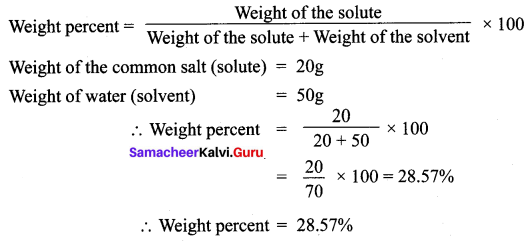VIII. HOT Questions.

Question 1.
Observe the diagram.

1. Which is a concentrated solution and why?
2. Which is dilute solution and why?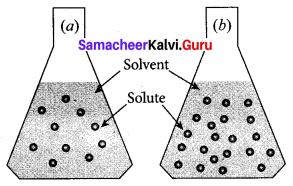1. Flask (b) is a concentrated solution. Because (b) flask contains a large number of solute particles than (a) flask.
2. Flask (a) is a dilute solution. Because (a) flask contains a lesser number of solute particles than (b) flask.

Question 2.
Where we use the phrase – “Like dissolves Like” and explain the meaning of the phrase?
The phrase “like dissolves like” is often used for predicting solubility. This expression means that dissolving occurs when similarities exist between the solvent and the solute. For example, Common salt is a polar compound and dissolves readily in polar solvent like water.

Question 3.
Why bubbling occurs when water is boiled?
The solubility of a gas in liquid decrease with increase in temperature. Generally, water contains dissolved oxygen. When water is boiled, the solubility of oxygen in water decreases, so oxygen escapes in the form of bubbles.

Question 4.
What happens when blue vitriol is heated? Explain.
When blue vitriol (Copper sulphate pentahydrate) crystals are gently heated, it loses it five water molecules and becomes colourless anhydrous copper sulphate.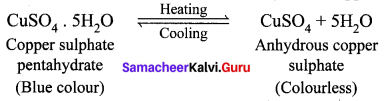### Samacheer Kalvi 10th Science Solutions Additional Problems Solved

Question 1.
Take 10 g of common salt and dissolve it in 40 g of water. Find the concentration of a solution in terms of weight per cent.
Solution:
Weight percent =  Weight of the solute  Weight of solute + Weight of solvent ×100
=1010+40×100=20%.

Question 2.
2 g of potassium sulphate was dissolved in 12.5 ml of water. On cooling, the first crystals appeared at 60°C. What is the solubility of potassium sulphate in water at 60°C? Solution. 12.5 ml of water weighs 12.5 g.
Solution:
In 12.5 g of water, the amount of potassium sulphate dissolved is 2 g.
In 1 g of water, the amount of potassium sulphate dissolved is 2 / 12.5 g.
Hence in 100 g of water, the amount of potassium sulphate dissolved is (2 × 100) / 12.5 = 16 g.
The solubility of potassium sulphate in the water at 60°C is 16 g.

Question 3.
50 g of a saturated solution of NaCl at 30°C is evaporated to dryness and 13.2 g of dry NaCl was obtained. Find the solubility of NaCl at 30°C in water.
Solution:
Mass of water in solution = 50 – 13.2 = 36.8 g
Solubility of NaCl
== Mass of NaCl Mass of water ×10013.236.8×100=36g
Solubility of NaCl = 36 g (approx).

Question 4.
An empty evaporating dish weighs 20.0 g. After adding a saturated solution of NaNO3, the dish weighs 66.0 g. When evaporated to dryness, the dish with crystals weighs 42.5 g. Find the solubility of NaNO3 at 20°C.
Solution:
Weight of saturated solution of NaNO3 = (66.0 – 20.0) g = 46.0 g
Weight of crystals of NaNO3 = (41.5 – 20.0) g = 21.5 g
Weight of water in saturated solution = (46.0 – 21.5) g = 24.5 g
Solubility of NaNO3 =  Weight of NaNO 3 crystals  Weight of water ×100
=21.524.5×100=87.7g
Solubility of NaNO3 at 20°C is = 87.7 g in 100 g H2O.

Question 5.
30 g sugar is dissolved in 170g of water. Calculate the concentration of solution in terms of weight percent.
Solution:
Weight percent =  Weight of the solute  Weight of solute + Weight of solvent ×100
=3030+170×10=15%.

Question 6.
50 g common salt is dissolved in 150 g of water; Find oat the concentration of solution in terms of weight percent.
Solution:
Weight percent =  Weight of the solute  Weight of solute + Weight of solvent ×100
=5050+150×100
= 25 %.

## How to Prepare using Samacheer Kalvi 10th Science Chapter 9 Solutions Notes PDF?

Students must prepare for the upcoming exams from Samacheer Kalvi 10th Science Chapter 9 Solutions Notes PDF by following certain essential steps which are provided below.

• Use Samacheer Kalvi 10th Science Chapter 9 Solutions notes by paying attention to facts and ideas.
• Pay attention to the important topics
• Refer TN Board books as well as the books recommended.
• Correctly follow the notes to reduce the number of questions being answered in the exam incorrectly
• Highlight and explain the concepts in details.

## Frequently Asked Questions on Samacheer Kalvi 10th Science Chapter 9 Solutions Notes

#### How to use Samacheer Kalvi 10th Science Chapter 9 Solutions Notes for preparation??

Read TN Board thoroughly, make separate notes for points you forget, formulae, reactions, diagrams. Highlight important points in the book itself and make use of the space provided in the margin to jot down other important points on the same topic from different sources.

#### How to make notes for Samacheer Kalvi 10th Science Chapter 9 Solutions exam?

Read from hand-made notes prepared after understanding concepts, refrain from replicating from the textbook. Use highlighters for important points. Revise from these notes regularly and formulate your own tricks, shortcuts and mnemonics, mappings etc.
Share: